#Reasoning Questions : Direction Sense Set 18

Hello Aspirants.

Welcome to Online Reasoning Section in AffairsCloud.com.Here we are creating Best question samples from Direction Sense test with explanation, which is common for IBPS, SBI, RBI, IPPB, LIC, SSC and other  competitive exams. We have included Some questions that are repeatedly asked in bank exams !!!

Direction

(Directions 1 -5): Read the following information carefully and answer the questions given below it:

Nine cars – A, B, C, D, E, F, G, H and I were located at some distance from each other in a parking area. C was 16 m \$ to that of B. A was 6 m % of that of B and H was 12 m # of that of A. G was 8 m @ of that of H while D was 24 m \$ of that of G and F was 12 m % of that of G. I was situated just in the middle of B and C while E was just in the middle of H and D.

Here, % means North, # means South, \$ means East and @ means West
Note: If two symbols are given simultaneously then both the directions will be considered.

For example,
X%\$Y means Y is to the North-East of X
Y%\$X means X is to the North-East of Y

1. Which of the following is/are true about B?
A. [email protected]%@F B. B#@G C. B#\$D
1) All are true
2) Both A and B
3) Only A
4) Both B and C
5) Only C
Answer – 1) All are true
Explanation :
Cars – A, B, C, D, E, F, G, H and I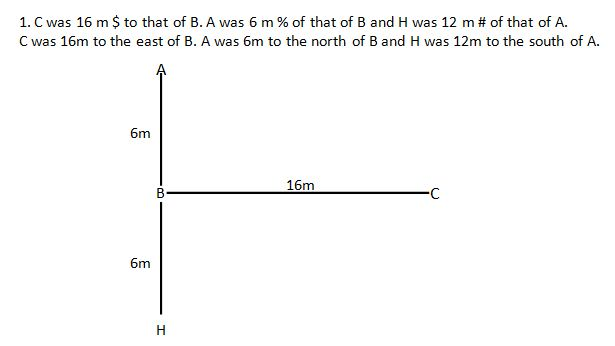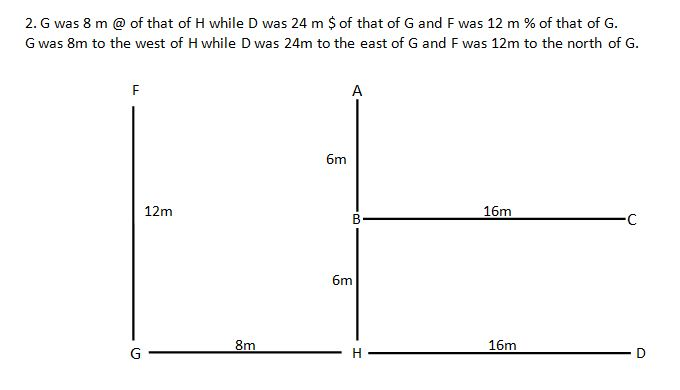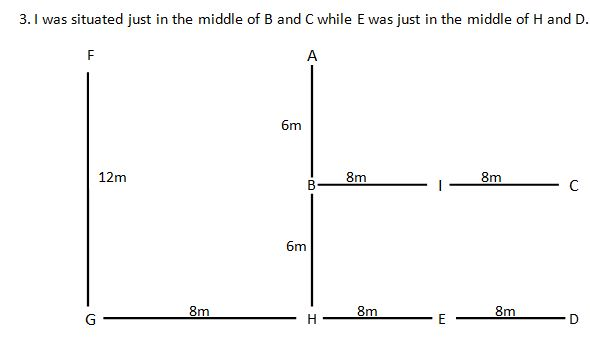A. B is west of I and F is north – west of B.
B. G is south – west of B.
C. D is south – east of B.
Hence, all are true.

2. What is the direction of D with respect to F?
1) South
2) South – west
3) North – east
4) North – west
5) South – east
Answer – 5) South – east

3. What is the shortest distance between car B and car F?
1) 12m
2) 10m
3) 9m
4) 11m
5) None of these

4. Which of the following cars lie in the straight line?
1) G and B
2) C and H
3) G and E
4) E and A
5) None of these
Answer – 3) G and E

5. What is the direction of E with respect to I?
1) South
2) South – west
3) North – east
4) North
5) None of these

(Directions 6 – 10): Read the following information carefully and answer the questions given below it:
6 dogs B, D, F, H, M and L are made to stand in a row facing north and distance between two adjacent dogs increases from left to right in consecutive integral multiple of 4.
Distance between F and H is 60m and only two dogs stand between them. L sits immediate left of F. B sits somewhere to the right of L and distance between them is in multiple of six. Distance between F and D is 44m.
Dog B starts moving towards east, after moving 12m, it turns to its right and moves 60m. From there after turning to its left and walking for a distance of 5m, it comes to halt at a point V.
Dog L moves in north direction for a distance of 20m and then turn to its left and moves 26m. After taking one more turn to its left it stops at a point W after walking 5m.

1. What is the distance between dogs H and F?
1) 50m
2) 70m
3) 40m
4) 60m
5) 30m
Solution:
Dogs – B, D, F, H, L and M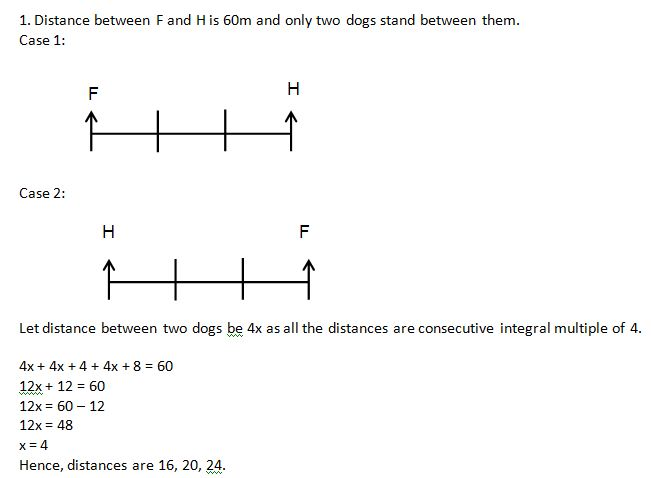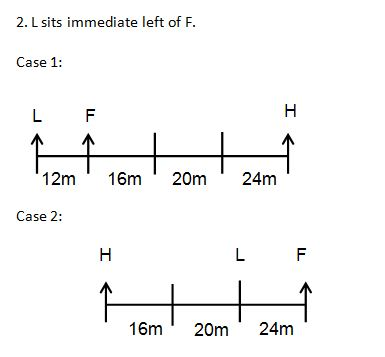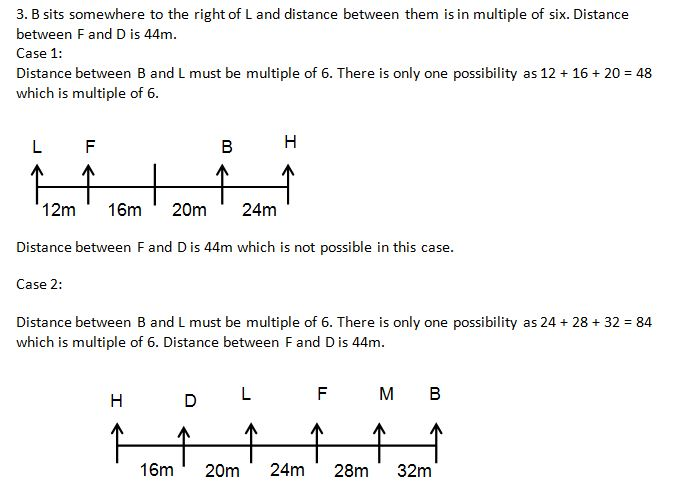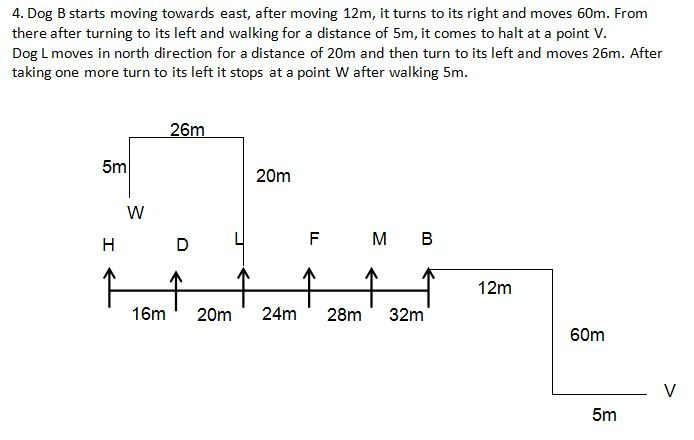2. In which direction dog B is facing now?
1) North
2) South
3) North-East
4) East
5) West

3. Who among the following is on the immediate right of D?
1) H
2) F
3) L
4) M
5) B

4. How many dogs are there between H and B?
1) None
2) One
3) Two
4) Three
5) Four